C code to implement Lexical Analyzer

C program to implement Lexical Analyzer

#include<stdio.h>
#include<string.h>
#include<stdlib.h>

void removeduplicate();
void final();
int Isiden(char ch);
int Isop(char ch);
int Isdel(char ch);
int Iskey(char * str);
void removeduplicate();

char op={'+','-','*','/','=','<','>','%'};
char del={'}','{',';','(',')','[',']',','};
char *key[]={"int","void","main","char","float"};

//char *operato[]={"+","-","/","*","<",">","=","%","<=",">=","++"};

int idi=0,idj=0,k,opi=0,opj=0,deli=0,uqdi=0,uqidi=0,uqoperi=0,kdi=0,liti=0,ci=0;
int uqdeli,uqopi,uqideni,l=0,j;
char uqdel,uqiden,uqop,keyword;
char iden,oper,delem,litral,lit,constant;

void lexanalysis(char *str)
{
int i=0;
while(str[i]!='\0')
{
if(Isiden(str[i]))     //for identifiers
{
while(Isiden(str[i]))
{
iden[idi][idj++]=str[i++];
}
iden[idi][idj]='\0';
idi++;idj=0;
}
else
if(str[i]=='"')         //for literals
{
lit[l++]=str[i];
for(j=i+1;str[j]!='"';j++)
{
lit[l++]=str[j];
}
lit[l++]=str[j];lit[l]='\0';
strcpy(litral[liti++],lit);
i=j+1;
}
else
if(Isop(str[i]))        // for operators
{
while(Isop(str[i]))
{
oper[opi][opj++]=str[i++];
}
oper[opi][opj]='\0';
opi++;opj=0;
}
else
if(Isdel(str[i]))     //for delemeters
{
while(Isdel(str[i]))
{
delem[deli++]=str[i++];
}
}
else
{
i++;
}
}

removeduplicate();
final();
}

int Isiden(char ch)
{
if(isalpha(ch)||ch=='_'||isdigit(ch)||ch=='.')
return 1;
else
return 0;
}

int Isop(char ch)
{
int f=0,i;
for(i=0;i<8&&!f;i++)
{
if(ch==op[i])
f=1;
}
return f;
}

int Isdel(char ch)
{
int f=0,i;
for(i=0;i<8&&!f;i++)
{
if(ch==del[i])
f=1;
}
return f;
}

int Iskey(char * str)
{
int i,f=0;
for(i=0;i<5;i++)
{
if(!strcmp(key[i],str))
f=1;
}
return f;
}

void removeduplicate()
{
int i,j;
for(i=0;i<20;i++)
{
uqdeli[i]=0;
uqopi[i]=0;
uqideni[i]=0;
}
for(i=1;i<deli+1;i++)  //removing duplicate delemeters
{
if(uqdeli[i-1]==0)
{
uqdel[uqdi++]=delem[i-1];
for(j=i;j<deli;j++)
{
if(delem[i-1]==delem[j])
uqdeli[j]=1;
}
}
}

for(i=1;i<idi+1;i++)  //removing duplicate  identifiers
{
if(uqideni[i-1]==0)
{
strcpy(uqiden[uqidi++],iden[i-1]);
for(j=i;j<idi;j++)
{
if(!strcmp(iden[i-1],iden[j]))
uqideni[j]=1;
}
}
}

for(i=1;i<opi+1;i++)  //removing duplicate  operators
{
if(uqopi[i-1]==0)
{
strcpy(uqop[uqoperi++],oper[i-1]);
for(j=i;j<opi;j++)
{
if(!strcmp(oper[i-1],oper[j]))
uqopi[j]=1;
}
}
}

}
void final()
{
int i=0;
idi=0;
for(i=0;i<uqidi;i++)
{
if(Iskey(uqiden[i]))        //identifying keywords
strcpy(keyword[kdi++],uqiden[i]);
else
if(isdigit(uqiden[i]))    //identifying constants
strcpy(constant[ci++],uqiden[i]);
else
strcpy(iden[idi++],uqiden[i]);
}

// printing the outputs

printf("\n\tDelemeter are : \n");
for(i=0;i<uqdi;i++)
printf("\t%c\n",uqdel[i]);

printf("\n\tOperators are : \n");
for(i=0;i<uqoperi;i++)
{
printf("\t");
puts(uqop[i]);
}

printf("\n\tIdentifiers are : \n");
for(i=0;i<idi;i++)
{
printf("\t");
puts(iden[i]);
}

printf("\n\tKeywords are : \n");
for(i=0;i<kdi;i++)
{
printf("\t");
puts(keyword[i]);
}

printf("\n\tConstants are :\n");
for(i=0;i<ci;i++)
{
printf("\t");
puts(constant[i]);
}

printf("\n\tLiterals are :\n");
for(i=0;i<liti;i++)
{
printf("\t");
puts(litral[i]);
}
}
void main()
{
char str;
//clrscr();
printf("\nEnter the string : ");
scanf("%[^\n]c",str);
lexanalysis(str);
//getch();
}

Output:-

Related Programs:-

Find out First and Follow in a given Grammar

Regular Grammar

SLR Parser

Context Free Grammar (CFG)

DFA (Deterministic Finite Automata)

1.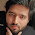isop should have prototype

2.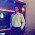Declare all function before lexicalanalysis() function.

3.using automata while implementing lexical analyser is much simple and easy to understand, i think this is a simple "checking" program ..... not an efficient one....

4.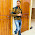Updated programs as feedback given by all above users...thanks to all.

1.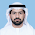2.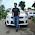Thanks Md Mohsin

3.5.This comment has been removed by the author.

6.can you make it read more than just one line? or like make it read from a txt file? that would be much appreciated

7.can you make it read more than just one line? or like make it read from a txt file? that would be much appreciated

8.This comment has been removed by the author.

9.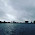isalpha and isdigit not works!

1.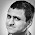This comment has been removed by the author.

2.This comment has been removed by the author.

3.include library ctype.h

10.not work

11.it work's for me!!

12.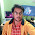You don't know a thing about lexical analyzer. why this stupid format of output? what use this can be in the later steps of the compilation?

1.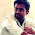Its just an implementation example.Not an actual lexical analyzer.

13.void lexanalysis(char *str)
i can not understand its operation and it

1.14.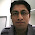Thanks for your code sample, It helps me a lot, cheers!!!!

15.I have been writing an argumentative essay with one company about coding. I used a lot of information from this website so ti is a grate source material :D Thank you so much for sharing all this :)

16.This is really interesting, You are a very skilled blogger. I've joined your rss feed and look forward to seeking more of your fantastic post. Also, I have shared your website in my social networks!
web design packages